Courses

# NEET Previous Year Questions (Level 1): Waves

## 14 Questions MCQ Test Physics For JEE | NEET Previous Year Questions (Level 1): Waves

Description
This mock test of NEET Previous Year Questions (Level 1): Waves for JEE helps you for every JEE entrance exam. This contains 14 Multiple Choice Questions for JEE NEET Previous Year Questions (Level 1): Waves (mcq) to study with solutions a complete question bank. The solved questions answers in this NEET Previous Year Questions (Level 1): Waves quiz give you a good mix of easy questions and tough questions. JEE students definitely take this NEET Previous Year Questions (Level 1): Waves exercise for a better result in the exam. You can find other NEET Previous Year Questions (Level 1): Waves extra questions, long questions & short questions for JEE on EduRev as well by searching above.
QUESTION: 1

### To demonstrate the phenomenon of interference we require two sources which emit radiations of   (AIEEE 2003)

Solution:

Sustained interference is possible with coherent sources only I.e. sources having the same frequency and constant or zero phase difference.

QUESTION: 2

### The maximum number of possible interference maxima for slit-separation equal to twice the wavelength in Young's double-slit experiment, is    (AIEEE 2004)

Solution:

For maxima, d sinθ =nλ
⇒2λsinθ=nλ
2sinθ=n
∴sinθ=n/2​
∵sinθ≤1
⇒n/2​≤1
n≤2
nmax​=2
n=0,1, 2,
So maximum number of possible maxima=5
n=0,±1,±2

QUESTION: 3

### When an unpolarized light of intensity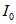is incident on a polarizing sheet, the intensity of the light which does not get transmitted is  (AIEEE 2005)

Solution:

As the intensity of an unpolarised light is Io therefore intensity of light transmitted from a polarizing sheet is Io/2
Hence intensity of light which doesn't get transmitted is Io - Io/2 = Io/2

QUESTION: 4

If I0 is the intersity of the principal maximum in the single slit diffraction pattern, then what will be its intensity when the slit width is doubled ?

(AIEEE 2005)

Solution:

When the slit width is doubled the energy falling per sec on the screen is doubled but angular width of central maxima (Δθ=2λ/ b ​) is halved. Hence intensity of the central maxima will be 4I0

QUESTION: 5

A Young's double slit experiment uses a monochromatic source. The shape of the interference fringes formed on a screen is

(AIEEE 2005)

Solution:

Monochromatic means light with single wavelength Hence shape of interference fringes formed on the screen is straight line. If instead of double slit experiment double hole experiment was given shape would have been hyperbolla.
Hence correct option is D , Straight line.

QUESTION: 6

In a Young's double slit experiment the intensity at a point where the path difference is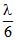(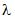being the wavelength of the light used) is I. If I0 denotes the maximum intensity,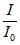is equal to

(AIEEE 2007)

Solution:
QUESTION: 7

A mixture of light, consisting of wavelength 590 nm and an unknown wavelength, illuminates Young's double slit and gives rise to two overlapping interference patterns on the screen. The central maximum of both lights coincide. Further, it is observed that the third bright fringe of known light coincides with the 4th bright fringe of the unknown light. From this data, the wavelength of the unknown light is

(AIEEE 2009)

Solution: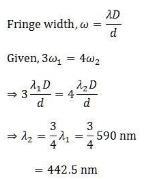QUESTION: 8

The initial shape of the wave front of the beam is

(AIEEE 2010)

Solution:

As the beam is initially parallel, the shape of the wave front is planar.

QUESTION: 9

This question has a paragraph followed by two statements, Statement I and Statement II. Of the given four alternatives after the statements, choose the one that describes the statements.A thin air film is formed by putting the convex surface of a plane-convex lens over a plane glass plate. With monochromatic light, this film gives an interference pattern due to light reflected from the top (convex) surface and the bottom (glass plate) surface of the file

Statement I : When light reflects from the air-glass plate interface, the reflected wave suffers a phase change or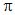.

Statement II : The centre of the inerference pattern is dark.

(AIEEE 2011)

Solution:

As the centre of the pattern is dark, we know that the phase difference is (2n+1)π
Furthermore, we also know that for the center of the interference pattern, there is no path difference. So the difference can only be due to reflection which is π
Therefore, both the assertion and the reason are correct and the reason is explanatory

QUESTION: 10

In a Young's double slit experiment, the two slits act as coherent source of waves of equal amplitude A and wavelength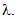In another experiment with the same arrangement the two slits are made to act as incoherent sources of waves of same amplitude and wavelength. If the intensity at the middle point of the screen in first case is I1 and in the second case I2, then the ratio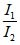is

( AIEEE 2011)

Solution: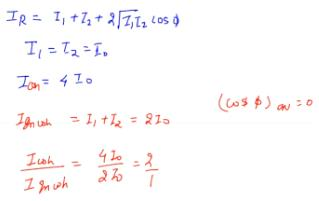QUESTION: 11

At two points P and Q on screen in Young's double slit expereiment, waves from slits S1 and S2 have a path difference of 0 and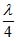respectively. The ratio of intensities at P and Q will be

(AIEEE 2011)

Solution:

At point 'P' intensity is maximum
∴IP​=4I
At point 'Q', phase difference, δ=2π​×λ/ λ x4​=π/2​
∴IQ​=4Icos2(δ/2​)
∴IQ​=4Icos2(π/4​)=4I×1/2​=2I
∴​IP/IQ ​​=2

QUESTION: 12

In Young's double slit experiment, one of the slit is wider than other, so that amplitude of the light from one slit is double of that from other slit. If Im be the maximum intensity, the resultant intensity I when very interfere at phase difference f, is given by

(AIEEE 2012)

Solution: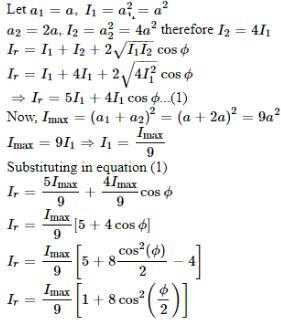QUESTION: 13

A beam of unpolarised light of intensity I0 is passed through a polaroid A and then through another polaroid B which is oriented so that its principal plane makes an angle of 45° relative to that of A. The intensity of the emergent light is :

(AIEEE 2013)

Solution: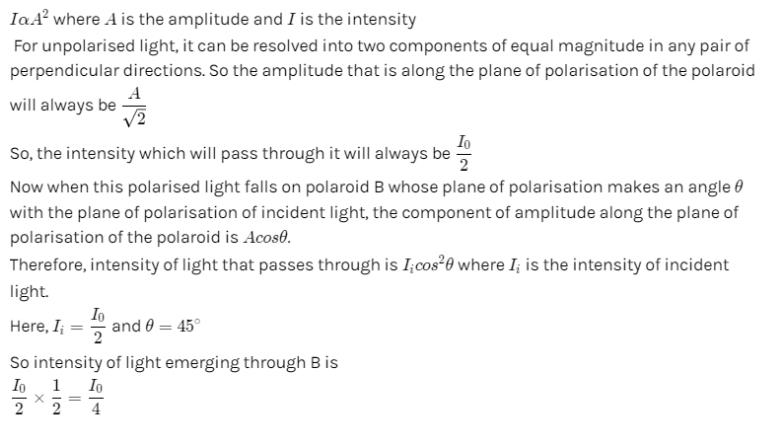QUESTION: 14

Two coherent point sources S1 and S2 are separated by a small distance 'd' as shown. The fringes obtained on the screen will be :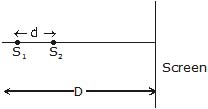(AIEEE 2013)

Solution: